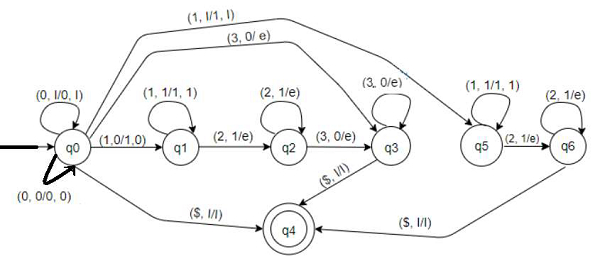# Construct Pushdown automata for L = {0n1m2m3n | m,n = 0} in C++

We are given with a language “L” and the task is to construct a pushdown automata for the given language which explains that the occurrences of 0’s and 3’s will be equal and occurrences of 1’s and 2’s will be equal and also occurrences of all the numbers should be minimum 1 which can also makes the string NULL and it should be accepted by the automata.

## What is pushdown Automata?

A pushdown automata or pushdown automaton or PDA is a technique to implement a context−free grammar in a similar way we design Deterministic Finite Automaton or DFA for a regular grammar. A DFA can operate on finite data, but a PDA can operate on infinite data. We can understand pushdown automata as the combination of “finite state machine” and a “stack”.

A pushdown automaton has three components −

• an input tape,

• a control unit, and

• a stack with infinite size.

A PDA can be formally described as a 7−tuple (Q, Σ, S, δ, q0, I, F) −

• Q is the finite number of states

• Σ is input alphabet

• S is stack symbols

• δ is the transition function: Q × (Σ υ {ε}) × S × Q × S*

• q0 is the initial state (q0 Ε Q)

• I is the initial stack top symbol (I Ε S)

• F is a set of accepting states (F Ε Q)

Let’s construct a pushdown Automata for the given languageThe strings that are acceptable by this PDA are of the form −

• 0n3n − 03, 0033, 000333 etc. No. of 0s is equal to no. of 3s. When m is 0 we will have no 1s and 2s. Keep pushing 0s and as soon as the first 3 is encountered then pop 0s. If we reach the end of the string and are left with no 0s then the string is accepted.

• 1m2m − 12, 1122, 111222 etc. No. of 1s is equal to no. of 2s. When n is 0 we will have no 0s and 3s. Keep pushing 1s and as soon as the first 2 is encountered then pop 1s. If we reach the end of the string and are left with no 1s then the string is accepted.

• 0n1m2m3n − 0123, 001233, 011223 etc. No. of 0s is equal to no. of 3s and 1s equal to 2s. Keep pushing 0s and 1s. As soon as the first 2 is encountered then pop 1s if it's on top and then pop 0s for the rest of 3s. If we reach the end and no 0 is left then the string is accepted.

• NULL string is also accepted. 00102030.

## Let’s understand the machine

• Transitions for state q0 −

• ( 0, I/0,I ) − If top of stack is I and current input symbol is 0 then push 0 to top of stack and remain at q0. Stack becomes 0I...

• ( 0, 0/0,0 ) − If top of stack is 0 and current input symbol is also 0 then push 0 to top of stack and remain at q0. Stack becomes 00.... Keep pushing 0s until the next 1 or 3.

• ( 1, 0/1,0 ) − If top of stack is 0 and current input symbol is 1 then push 1 to top of stack and move to q1. Stack becomes 10...

• ( 1, I/1,I ) − If top of stack is I and current input symbol is 1 then push 1 and move to q5.

• ( 3, 0/e ) − If top of stack is 0 and current input symbol is 3 then pop 0 and move to q3.

• ( $, I/I ) − If top of stack is I and there is no input then do nothing and move to q4. For NULL string. • Transitions for state q1 − • ( 1, 1/11 ) − If top of stack is 1 and current input symbol is also 1 then push 1 to top of stack and remain at q1. Stack becomes 11.... Keep pushing 1s until the next 2. • ( 2, 1/e ) − If top of stack is 1 and current input symbol is 2 then pop 1 and move to q2. • Transitions for state q2 − • ( 2, 1/e ) − If top of stack is 1 and current input symbol is 2 then pop 1 and remain at q2. • ( 3, 0/e ) − If top of stack is 0 and current input symbol is 3 then pop 0 and move to q3. • Transitions for state q3 − • ( 3, 0/e ) − If top of stack is 0 and current input symbol is 3 then pop 1 and remain at q3. • ($, I/I ) − If top of stack is I and there is no input then do nothing and move to q4. For NULL string.

• Transitions for state q5 −

• ( 1, 1/1,1 ) − If top of stack is 1 and current input symbol is also 1 then push 1 to top of stack and remain at q5. Stack becomes 11.... Keep pushing 1s until the next 2.

• ( 2, 1/e ) − If top of stack is 1 and current input symbol is 2 then pop 1 and move to q6.

• Transitions for state q6 −

• ( 2, 1/e ) − If top of stack is 1 and current input symbol is 2 then pop 1 and remain at q6.

• ( \$, I/I ) − If top of stack is I and there is no input then do nothing and move to q4. For NULL string.